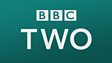Bill and Bernie use labelled balloons to show subtractions of 10 from several two-digit numbers which are less than 100. Bernie thinks of a number and Bill must subtract ten from that number.This clip is from:
Numbertime, Addition and Subtraction: Subtracting one from another